Logic Chapter Seven
Next Chapter

Indirect Proof

The logical relationship that we call "validity" can exist between a set of premises and an obviously false conclusion, if you choose the right kind of set of premises. Remember this argument?

 Elvis is dead. Elvis is alive. Laura Schlessinger is a woolly mammoth. De ~De Wl Dx: x is dead Wx: x is a wooly Mammoth e: Elvis l: Laura Schlessinger

Remember that for the argument to be invalid, it has to be possible for the premises to all be true at the same time the conclusion is false. Since the premises can't all be true, the argument is valid. In fact, any argument in which the premises cannot all be true is valid, no matter how blatantly false the conclusion.  Since this argument is valid, we should be able to prove it with a derivation, and that means we should have a rule that will let us successfully complete a derivation for this valid argument form.

 Rule 5. Indirect Proof (Indicated by "IP") (This rule has two parts) Part One: Whenever you write a show line for any formula, you can write the exact negation of that formula, provided that it is the very next thing you write. (Indicate by writing AIP on that line) Part Two: If any formula and its negation both appear as available lines in a derivation without an uncancelled "show" between them, then the closest "show" that is above them both may be cancelled, and a box may be drawn around the closest one and any other lines there may be between it and that show line. If the relevant show line is line #1, then the derivation is successfully finished. (Put IP by the "show") (The first six rules are conveniently listed on Logic Rules Sheet One)

Now, let's give it a go. As always, we start by writing the conclusion after the word "show."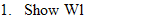Now, we could write to negation of "Wl" as line 2, but we don't actually need to, so I'm not going to bother. Instead, I'm just going to write in one premise.And then the other. (The order doesn't matter.)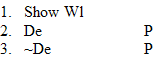Since we have our contradiction, we can box and cancel under indirect proof. I add the letters "IP" to show we used Indirect Proof.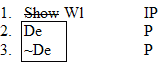And there we are.

Here's a more complicated example.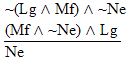Now one way to think about deriving an argument like this is to ask yourself what kind of contradiction you can make here. Notice that the premises are both conjunctions, and that if we simplified them both we would get the following terms: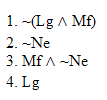Can we see any contradictions here? No, none of these terms contradict each other. But one of them is a conjunction, so we could imagine what we would get if we simplified it.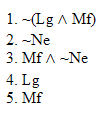Hmmm, still no contradictions. And we can't simplify any more. I know! Let's try conjoining the smaller terms. That'll be fun. (No it won't.) So we get: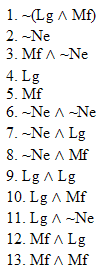Holy cr ... That's a lot of terms. Any contradictions here. No ... no ... Wait! Look! Number 1 and number 10 contradict each other. So now all we have to do is get those two together, and we'll have it!

Okay, so we start in the usual way.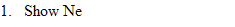Since we're getting everything out of our premises, we don't need to do an Assumption for Indirect Proof, so we'll just write in a premise.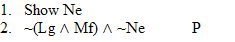And the other premise.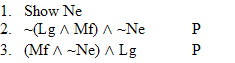Now we need Mf and Lg to make that conjunction. Let's get Mf first. Well, we have to get the conjunction it's a part of first, so lets do that.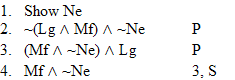Now we can get Mf. (Can you figure out why we couldn't do it straight away?) But for some reason I decided to get Lg first. I don't know why I did it this way, but it's okay.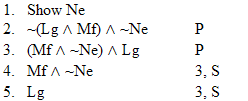Okay, let's get Mf this time.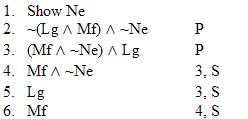And finally, at long last, we can make that conjunction!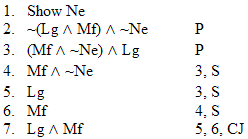Aaaaaaaaah! Oh wait, we're not finished! We need a contradiction, so lets get ~(Lg ^ Mf) out of that first premise.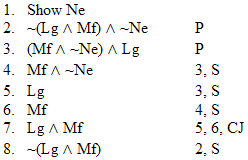Now we have a contradiction! So we can box and cancel.Finished!

Now test yourself. Try to derive each of the following arguments. If you can't solve the problem by yourself, struggle with it for a while, and if that doesn't work, click on the relevant argument for some hints.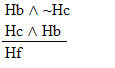Practice 7. Use your own paper or the answer sheet at practice. For each of the following arguments, determine whether it is valid or invalid. If it's valid prove it valid by DERIVATION. If it's invalid prove it invalid by venn diagram or truth table. (Try doing AIP as your first line.)
 1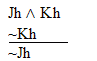234(Try doing AIP as your first line.)

Next Chapter